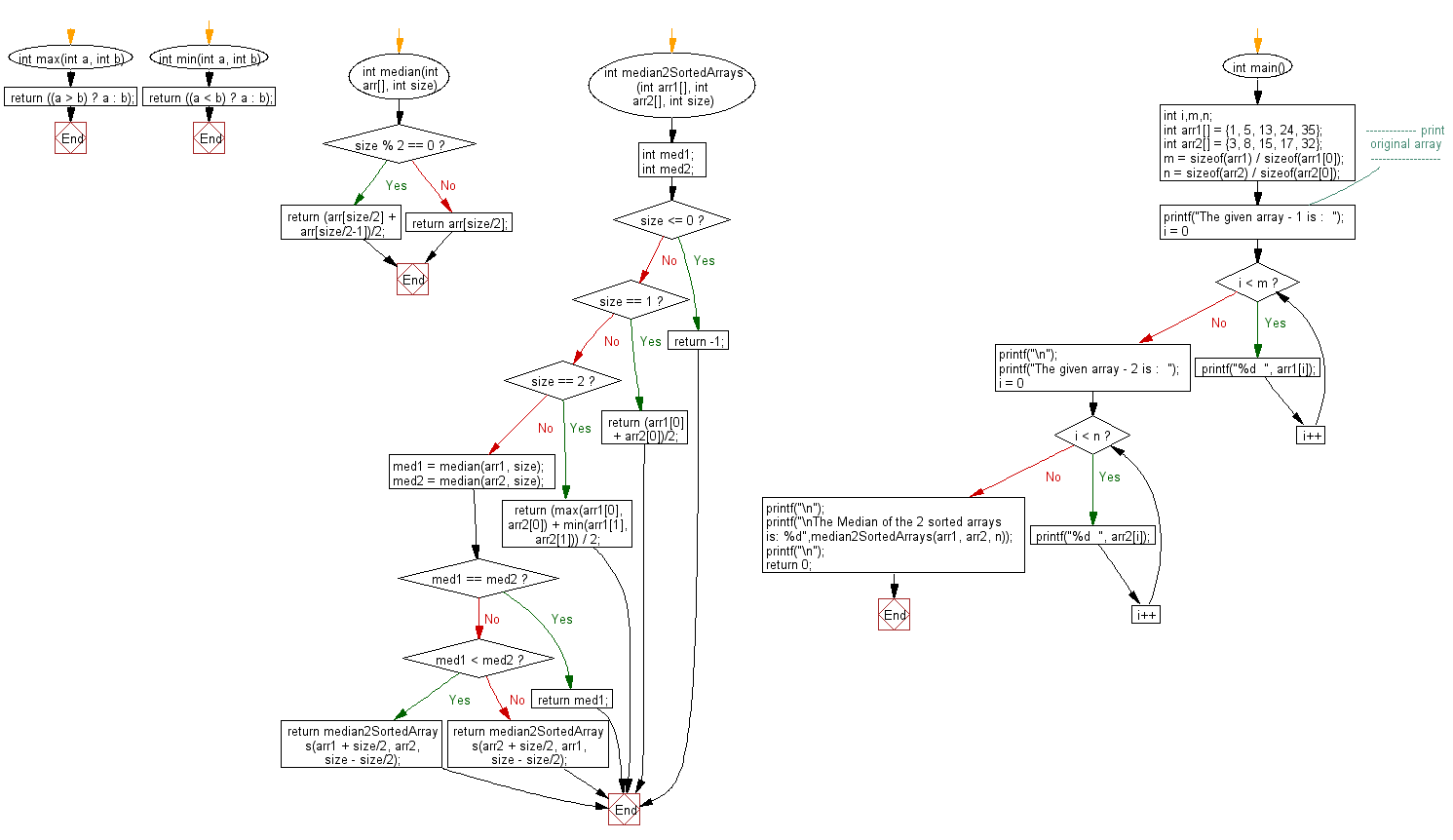﻿ C exercises: Find the median of two sorted arrays of same size - w3resource# C Exercises: Find the median of two sorted arrays of same size

## C Array: Exercise-64 with Solution

Write a program in C to find the median of two sorted arrays of same size.

Sample Solution:

C Code:

``````#include <stdio.h>

int max(int a, int b)
{
return ((a > b) ? a : b);
}
int min(int a, int b)
{
return ((a < b) ? a : b);
}
int median(int arr[], int size)
{
if (size % 2 == 0)
return (arr[size/2] + arr[size/2-1])/2;
else
return arr[size/2];
}
int median2SortedArrays(int arr1[], int arr2[], int size)
{
int med1;
int med2;
if(size <= 0) return -1;
if(size == 1) return (arr1 + arr2)/2;
if (size == 2) return (max(arr1, arr2) + min(arr1, arr2)) / 2;

med1 = median(arr1, size);
med2 = median(arr2, size);

if(med1 == med2) return med1;

if (med1 < med2)
{
return median2SortedArrays(arr1 + size/2, arr2, size - size/2);
}
else
{
return median2SortedArrays(arr2 + size/2, arr1, size - size/2);
}
}

int main()
{
int i,m,n;
int arr1[] = {1, 5, 13, 24, 35};
int arr2[] = {3, 8, 15, 17, 32};
m = sizeof(arr1) / sizeof(arr1);
n = sizeof(arr2) / sizeof(arr2);
//------------- print original array ------------------
printf("The given array - 1 is :  ");
for(i = 0; i < m; i++)
{
printf("%d  ", arr1[i]);
}
printf("\n");
//------------------------------------------------------
printf("The given array - 2 is :  ");
for(i = 0; i < n; i++)
{
printf("%d  ", arr2[i]);
}
printf("\n");
//------------------------------------------------------
printf("\nThe Median of the 2 sorted arrays is: %d",median2SortedArrays(arr1, arr2, n));
printf("\n");
return 0;
}
```
```

Sample Output:

```The given array - 1 is :  1  5  13  24  35
The given array - 2 is :  3  8  15  17  32

The Median of the 2 sorted arrays is: 14
```

Flowchart:C Programming Code Editor:

Improve this sample solution and post your code through Disqus.

What is the difficulty level of this exercise?

﻿